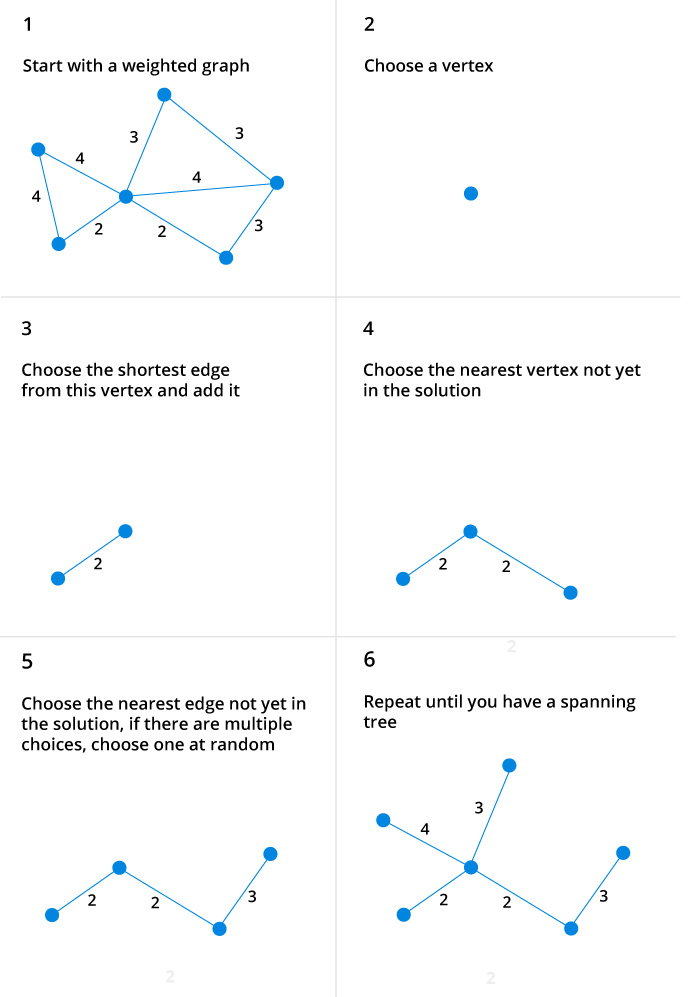# Prim's Algorithm

Prim's algorithm is a minimum spanning tree algorithm that takes a graph as input and finds the subset of the edges of that graph which

• form a tree that includes every vertex
• has the minimum sum of weights among all the trees that can be formed from the graph

## How Prim's algorithm works

It falls under a class of algorithms called greedy algorithms which find the local optimum in the hopes of finding a global optimum.

We start from one vertex and keep adding edges with the lowest weight until we we reach our goal.

The steps for implementing Prim's algorithm are as follows:

1. Initialize the minimum spanning tree with a vertex chosen at random.
2. Find all the edges that connect the tree to new vertices, find the minimum and add it to the tree
3. Keep repeating step 2 until we get a minimum spanning tree

## Example of Prim's algorithm## Prim's Algorithm pseudocode

The pseudocode for prim's algorithm shows how we create two sets of vertices U and V-U. U contains the list of vertices that have been visited and V-U the list of vertices that haven't. One by one, we move vertices from set V-U to set U by connecting the least weight edge.

``````T = ∅;
U = { 1 };
while (U ≠ V)
let (u, v) be the lowest cost edge such that u ∈ U and v ∈ V - U;
T = T ∪ {(u, v)}
U = U ∪ {v}``````

## Prim's Algorithm Implementation in C++

The program below implements Prim's algorithm in C++.  Although adjacency matrix representation of graph is used, this algorithm can also be implemented using Adjacency List to improve its efficiency.

``````#include <iostream>
#include <cstring>
using namespace std;

#define INF 9999999

// number of vertices in grapj
#define V 5

// create a 2d array of size 5x5
//for adjacency matrix to represent graph

int G[V][V] = {
{0, 9, 75, 0, 0},
{9, 0, 95, 19, 42},
{75, 95, 0, 51, 66},
{0, 19, 51, 0, 31},
{0, 42, 66, 31, 0}
};

int main () {

int no_edge;            // number of edge

// create a array to track selected vertex
// selected will become true otherwise false
int selected[V];

// set selected false initially
memset (selected, false, sizeof (selected));

// set number of edge to 0
no_edge = 0;

// the number of egde in minimum spanning tree will be
// always less than (V -1), where V is number of vertices in
//graph

// choose 0th vertex and make it true
selected = true;

int x;            //  row number
int y;            //  col number

// print for edge and weight
cout << "Edge" << " : " << "Weight";
cout << endl;
while (no_edge < V - 1) {

//For every vertex in the set S, find the all adjacent vertices
// , calculate the distance from the vertex selected at step 1.
// if the vertex is already in the set S, discard it otherwise
//choose another vertex nearest to selected vertex  at step 1.

int min = INF;
x = 0;
y = 0;

for (int i = 0; i < V; i++) {
if (selected[i]) {
for (int j = 0; j < V; j++) {
if (!selected[j] && G[i][j]) { // not in selected and there is an edge
if (min > G[i][j]) {
min = G[i][j];
x = i;
y = j;
}

}
}
}
}
cout << x <<  " - " << y << " :  " << G[x][y];
cout << endl;
selected[y] = true;
no_edge++;
}

return 0;
}``````

On running the above code, we get the output as:

```Edge : Weight
0 - 1 :  9
1 - 3 :  19
3 - 4 :  31
3 - 2 :  51```

## Prim's vs Kruskal's Algorithm

Kruskal's algorithm is another popular minimum spanning tree algorithm that uses a different logic to find the MST of a graph. Instead of starting from an vertex, Kruskal's algorithm sorts all the edges from low weight to high and keeps adding the lowest edges, ignoring those edges that create a cycle.